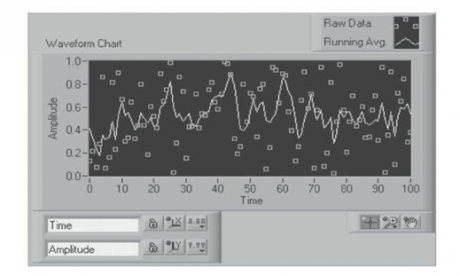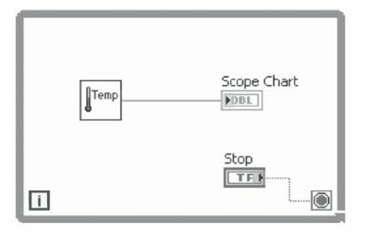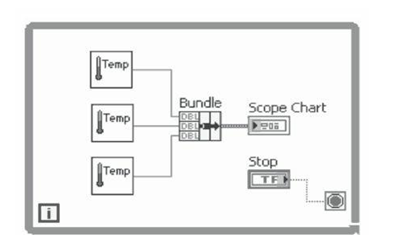# Waveform Charts In Labview

Rating: 5

13192

The WAVEFORM chart is a special type of numeric indicator that displays one or more plots of data typically acquired at a constant rate.
Waveform charts can display single or multiple plots. The below Figure shows the elements of a multiplot waveform chart. Two plots are displayed: Raw Data and Running Avg. The waveform chart maintains a history of data or buffers from previous updates.

If you would like to Enrich your career with an Android certified professional, then visit Mindmajix - A Global online training platform: “LabVIEW training” Course.This course will help you to achieve excellence in this domain.## Displaying A Single Plot On Waveform Charts

You can wire a scalar output directly to a waveform chart. The waveform chart terminal shown below Figure matches the input data type. If you pass the chart a single value or multiple values at a time, LABVIEW interprets the data as points on the chart and increments the x index by one starting at x = 0. The chart treats these inputs as new data for a single plot. The waveform chart accepts the WAVEFORM DATA TYPE which carries the data, start time, and delta t of a waveform. Use the Build Waveform function to plot time on the x-axis of the chart and automatically use the correct interval between markers on the x-scale of the chart. A waveform that specifies t0 and a single-element Y array is useful for plotting data that is not evenly sampled because each data point has its own timestamp.## Displaying Multiple Plots On Waveform Charts

Waveform charts can display multiple plots together using the Bundle function located on the CLUSTER palette. In the below Figure, the Bundle function bundles the outputs of the three VIs to plot on the waveform chart. The waveform chart terminal changes to match the output of the Bundle function. To add more plots, use the positioning tool to resize the Bundle function.To pass data for multiple plots to a waveform chart, you can bundle the data together into a cluster of scalar numeric values, where each numeric represents a single point for each of the plots. If you want to pass multiple points per plot in a single update, wire an ARRAY of clusters of numeric values to the chart. Each numeric represents a single y value point for each of the plots. You can use the waveform data type to create multiple plots on a waveform chart. Use the Build Waveform function to plot time on the x-axis of the chart and automatically use the correct interval between markers on the x-scale of the chart. A 1D ARRAY of waveforms that each specifies t0 and a single-element Y array is useful for plotting data that is not evenly sampled because each data point has its own timestamp.If you cannot determine the number of plots you want to display until run time, or you want to pass multiple points for multiple plots in a single update, wire a 2D ARRAY of numeric values or waveforms to the chart. By default, the waveform chart treats each column in the array as a single plot. Wire a 2D array data type to the chart, right-click the chart and select Transpose Array from the shortcut menu to treat each row in the array as a single plot.Course Schedule
NameDates
LabVIEW TrainingJun 28 to Jul 13
LabVIEW TrainingJul 02 to Jul 17
LabVIEW TrainingJul 05 to Jul 20
LabVIEW TrainingJul 09 to Jul 24
Last updated: 26 June 2022